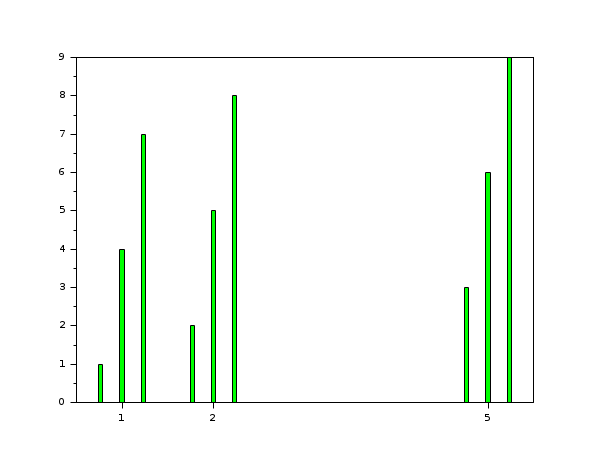Scilab Home page | Wiki | Bug tracker | Forge | Mailing list archives | ATOMS | File exchange
Scilab-Branch-6.1-GIT
Change language to: English - Français - Português - 日本語 -

# bar

bar histogram

### Syntax

```bar(y)
bar(x, y)
bar(x, y, width, colors, style)
bar(x, y [,width] [,colors] [,style])
bar(h, ..)```

### Arguments

h

an axes handle, (default: h=gca() ).

y

scalar, vector of size N, or matrix N*M of real numbers or encoded integers, with

• N : number of groups (each group of bars gathered on/around an x position)
• M : number of categories in each group

x

a real scalar or a vector of size N. By default,

• If `y` is a vector: `x=1:length(y)`
• If `y` is a matrix: `x=1:size(y,"r")`

width

(optional), a real scalar, defines the width (a percentage of the available room) for the bar (default: 0.8, i.e 80%).

colors

(optional) Single string or vector of M strings: colors names (among predefined ones) or "#RRGGBB" hexadecimal codes of categories colors. Default = default plot() colors series, starting with "blue".

style

a string, 'grouped' (default), or 'stacked'.

### Description

`bar(y,...) :` if y is a vector, then bar() draws a polyline which has the `polyline_style` type 6, versus x=1:length(y). If y is a matrix N*M, bar() draws M polylines (type 6), versus x=1:size(y,1). Each polyline represents a category, with a specific color.

`bar(x,y,...) :` if y is a vector, then bar() draws a polyline which has the `polyline_style` type 6, where x length = y length. If y is a matrix NxM then bar function draws M polylines which have the type 6. Each polyline corresponds to a column of y versus vector x.

`bar(h,...) :` specifies the targeted axes where the drawing is performed.

`bar(...,width,...) :` defines the relative width of the bar(s): 0<width<=1.

`bar(...,style,...) :` defines how the bar is drawn. If y is a matrix N*M (so M polylines of type 6), then there are two ways to draw the M bars. the style option = 'grouped' allows to center the M polylines versus each components of x, and the style option 'stacked' allows to stack them.

`bar(...,colors,...) :` defines the colors identifying categories and filling the corresponding subsets of bars.

If there are several bar() calls, the `barhomogenize` function allows to homogenize the width and style of all bars (i.e polylines of type 6) included in the current working axes.

### Examples

```// First example: draw a bar (i.e a polyline with polyline_style type =6) with
// width=0.5 and colors='yellow' and default style='grouped', x=1:length(y).
scf(0);
y=[1 -3 5];
bar(y,0.5,'yellow');``````// Second example: draw 3 bars (i.e 3 polylines with polyline_style type =6),default style='grouped'
scf(1);
x=[1 2 5];
y=[1  -5 6;3 -2 7;4  -3 8];
bar(x,y);``````// Third example : style='stacked'.
scf(2);
x=[1 2 5];
y=[1 4 7;2 5 8;3 6  9];
bar(x,y,'stacked');``````// Fourth example: width=0.2;colors='green'; default style='grouped'
scf(3);
x=[1 2 5];
y=[1 4 7;2 5 8;3 6 9];
bar(x,y,0.2,'green');```### History

 Версия Описание 6.0.1 A vector of M colors can now be provided, one for each category. Available colors are no longer restricted to the 10 major colors. All the predefined colors names can now be used. Colors can now be specified also by their "#RRGGBB" hexadecimal codes.